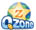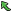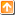## 2406: Power StringsTime Limit(Common/Java):3000MS/30000MS     Memory Limit:65536KByte
Total Submit: 2077            Accepted:1226

Description

Given two strings a and b we define a*b to be their concatenation. For example, if a = "abc" and b = "def" then a*b = "abcdef". If we think of concatenation as multiplication, exponentiation by a non-negative integer is defined in the normal way: a^0 = "" (the empty string) and a^(n+1) = a*(a^n).

Input

Each test case is a line of input representing s, a string of printable characters. The length of s will be at least 1 and will not exceed 1 million characters. A line containing a period follows the last test case.

Output

For each s you should print the largest n such that s = a^n for some string a.

Sample Input

Sample Output

Hint

This problem has huge input, use scanf instead of cin to avoid time limit exceed.

Source

Waterloo Jun.1 2002

|Back |   |Top|